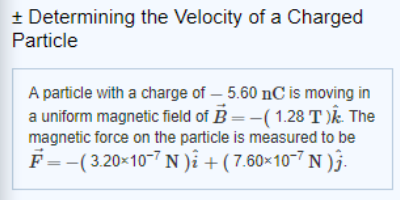# Problem: A particle with a charge of -5.60 nC is moving on a uniform magnetic field B = - (1.28 T)k. The magnetic force on the particle is measure to be F = -(3.20x10-7 N)I + (7.60x10-7 N)j.part A. Are there components of the velocity that cannot be determined by measuring force.part B. calcaluate the x component of the velocity of the partcile ( 3sig figs)part C. calcualte the y component of the velocity of the paricle ( 3sig Figs)part D. what is the angle between V and F give your answers in degrees ( 3sig figs)

###### FREE Expert Solution

Magnetic force on a moving charge is expressed as:

$\overline{){\mathbf{F}}{\mathbf{=}}{\mathbf{q}}{\mathbf{\left(}}{\mathbf{v}}{\mathbf{×}}{\mathbf{B}}{\mathbf{\right)}}}$

82% (344 ratings)###### Problem Details

A particle with a charge of -5.60 nC is moving on a uniform magnetic field B = - (1.28 T)k. The magnetic force on the particle is measure to be F = -(3.20x10-7 N)I + (7.60x10-7 N)j.

part A. Are there components of the velocity that cannot be determined by measuring force.

part B. calcaluate the x component of the velocity of the partcile ( 3sig figs)

part C. calcualte the y component of the velocity of the paricle ( 3sig Figs)

part D. what is the angle between V and F give your answers in degrees ( 3sig figs)